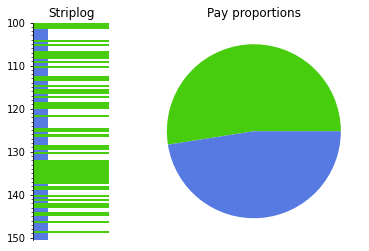# How to work with binary logs#

We will invent a binary log – maybe you can load it from an LAS file with welly.

import numpy as np
%matplotlib inline
import matplotlib.pyplot as plt

fake_depth = np.linspace(100, 150, 101)
fake_log = np.array([np.random.choice([0, 1]) for _ in fake_depth])

plt.figure(figsize=(15, 1))
plt.plot(fake_depth, fake_log, 'o-')

[<matplotlib.lines.Line2D at 0x7f9162986c80>]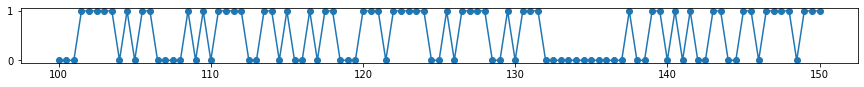## Make a striplog#

A Striplog is a sequence of Interval objects (representing a layer). Each Interval must contain a Component (representing the layer, perhaps a rock).

from striplog import Striplog, Component

comps = [
Component({'pay': True}),
Component({'pay': False})
]

s = Striplog.from_log(fake_log, cutoff=0.5, components=comps, basis=fake_depth)

s[-1].base.middle = 150.5 # Adjust the bottom thickness... not sure if this is a bug.


Each Interval in the striplog looks like:

s

top100.0
primary
 pay True
summary1.50 m of True
description
data
base101.5

## Plot the intervals#

To plot we need a legend, but we can generate a random one. This maps each Component to a colour (and a width and hatch, if you want).

We can generate a random legend:

from striplog import Legend

legend = Legend.random(comps)
legend.get_decor(comps[-1]).width = 0.2
legend.plot()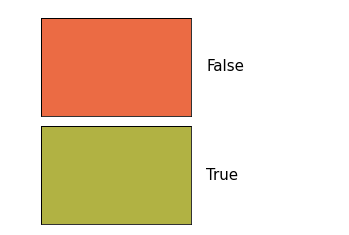Or we can make one with a bit more control:

legend_csv = """colour,hatch,width,component pay
#48cc0e,None,1,True
#5779e2,None,0.2,False"""

legend = Legend.from_csv(text=legend_csv)

legend.plot()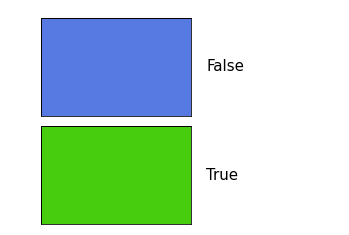s.plot(legend=legend, aspect=5)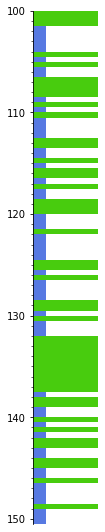## Remove thin things#

We can remove thin intervals:

pruned = s.prune(limit=1.0, keep_ends=True)


Now we can anneal the gaps:

annealed = pruned.anneal()


Then merge the adjacent intervals that are alike…

merged = annealed.merge_neighbours()  # Anneal works on a copy


We could have chained these commands:

merged = s.prune(limit=1.0, keep_ends=True).anneal().merge_neighbours()


Let’s plot all these steps, just for illustration:

fig, axs = plt.subplots(ncols=4, figsize=(6, 10))

axs = s.plot(legend=legend, ax=axs, lw=1, aspect=5)
axs.set_title('Original')
axs = pruned.plot(legend=legend, ax=axs, lw=1, aspect=5)
axs.set_yticklabels([])
axs.set_title('Pruned')
axs = annealed.plot(legend=legend, ax=axs, lw=1, aspect=5)
axs.set_yticklabels([])
axs.set_title('Annealed')
axs = merged.plot(legend=legend, ax=axs, lw=1, aspect=5)
axs.set_yticklabels([])
axs.set_title('Merged')
plt.show()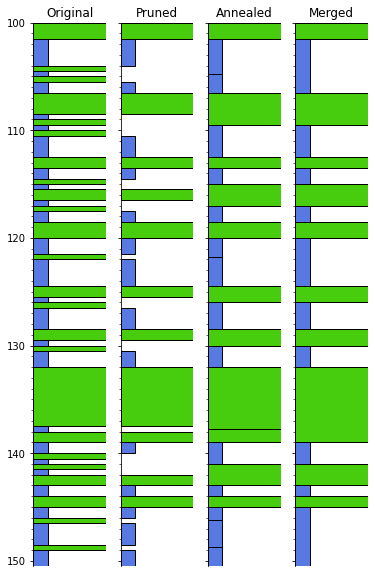## Dilate and erode#

This would be a binary thing, at least for now. I made an issue for this: https://github.com/agile-geoscience/striplog/issues/95

You need to be on striplog v 0.8.1 at least for this to work.

fig, axs = plt.subplots(ncols=4, figsize=(6, 10))

opening = s.binary_morphology('pay', 'opening', step=0.1, p=7)
closing = s.binary_morphology('pay', 'closing', step=0.1, p=7)

axs = s.plot(legend=legend, ax=axs, lw=1, aspect=5)
ntg = s.net_to_gross('pay')
axs.set_title(f'Original\n{ntg:.2f}')
axs = merged.plot(legend=legend, ax=axs, lw=1, aspect=5)
axs.set_yticklabels([])
ntg = merged.net_to_gross('pay')
axs.set_title(f'PAM\n{ntg:.2f}')  # Prune-anneal-merge
axs = opening.plot(legend=legend, ax=axs, lw=1, aspect=5)
axs.set_yticklabels([])
ntg = opening.net_to_gross('pay')
axs.set_title(f'Opening\n{ntg:.2f}')
axs = closing.plot(legend=legend, ax=axs, lw=1, aspect=5)
axs.set_yticklabels([])
ntg = closing.net_to_gross('pay')
axs.set_title(f'Closing\n{ntg:.2f}')
plt.show()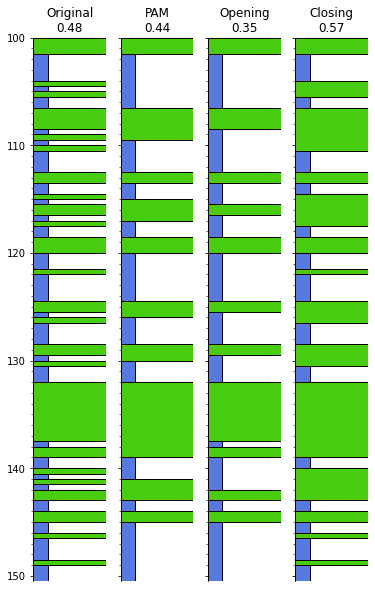## Some statistics#

We can get the unique components and their thicknesses:

s.unique

[(Component({'pay': False}), 26.5), (Component({'pay': True}), 24.0)]


We can get at the thickest (and thinnest, with .thinnest()) intervals:

s.thickest()

top132.0
primary
 pay True
summary5.50 m of True
description
data
base137.5

These functions optionally take an integer argument n specifying how many of the thickest or thinnest intervals you want to see. If n is greater than 1, a Striplog object is returned so you can see the positions of those items:

s.thickest(5).plot(legend=legend, lw=1, aspect=5)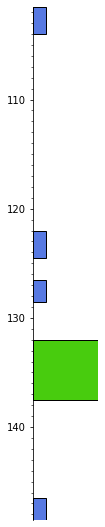## Bar plots and histograms#

We can make a bar plot of the layers:

s.bar(legend=legend)

<AxesSubplot:ylabel='Thickness'>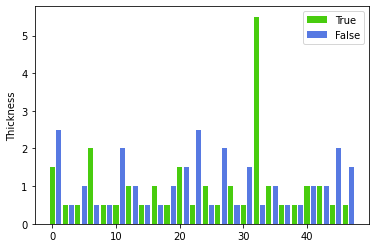More interesting is to sort the thicknesses:

s.bar(legend=legend, sort=True)

<AxesSubplot:ylabel='Thickness'>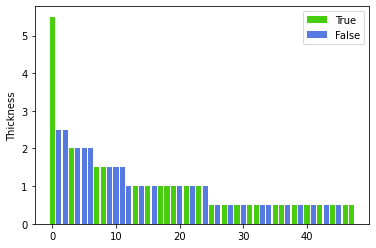Finally, we can make a thickness histogram of the various types of component present in the log.

n, ents, ax = s.hist(legend=legend)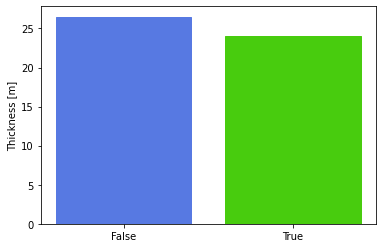s

Striplog(48 Intervals, start=100.0, stop=150.5)

legend

componentwidthhatchcolour
 pay True
1.0None#48cc0e
 pay False
0.2None#5779e2
data = [c for c in s.unique]
colors = [c['_colour'] for c in legend.table]

fig, axs = plt.subplots(ncols=2,
gridspec_kw={'width_ratios': [1, 3]})

axs = s.plot(ax=axs, legend=legend)
axs.set_title("Striplog")

axs.pie(data, colors=colors)
axs.set_title("Pay proportions")

plt.show()